# In the circuit shown in the figure, find the value of the current shown in the ammeter A.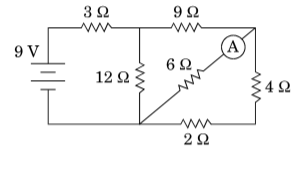figure 1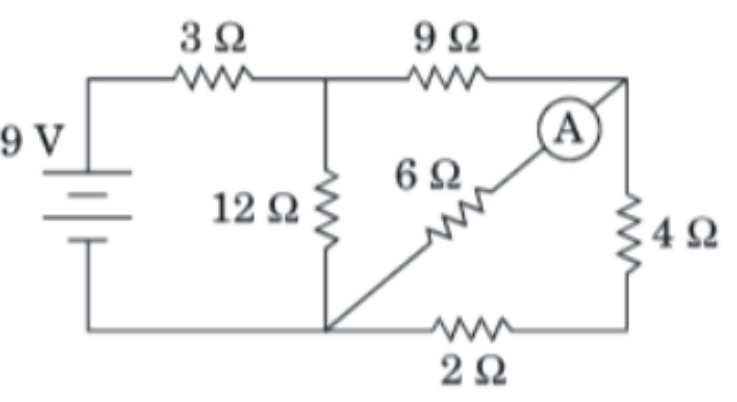to find the current shown in ammeter, find the current through 6 resistance. Here assume ammeter is ideal, so resistance of ammeter is is zero. The 4 resistance and 2 resisatnce are in series.

We know at series connection

parallel connection

So

The figure can be redrawn into

figure 2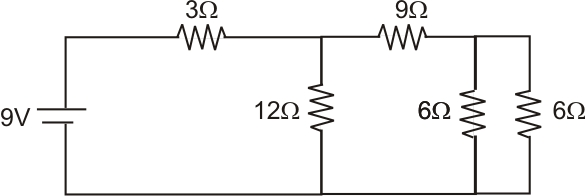and  are parallel so effective resistance is

Then figure become

figure 3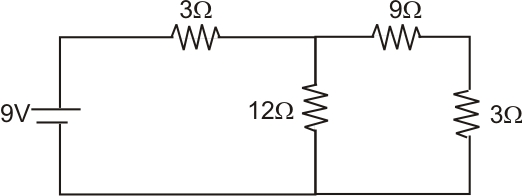9 and 3 are in series,

effective resistance is 9  + 3  = 12

Figure become,

figure 4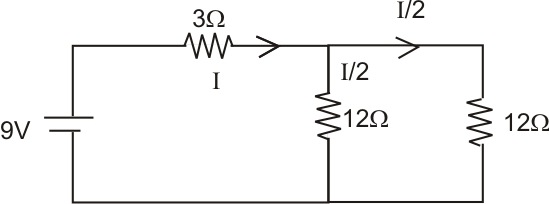12  and 12  are in parallel so,

figure 5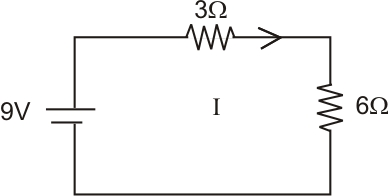3 and 6  are in series, so

3 + 6 = 9

By ohm's law,

In figure 4 both 12  resistance have  current = 0.5A

The equivalent figure is,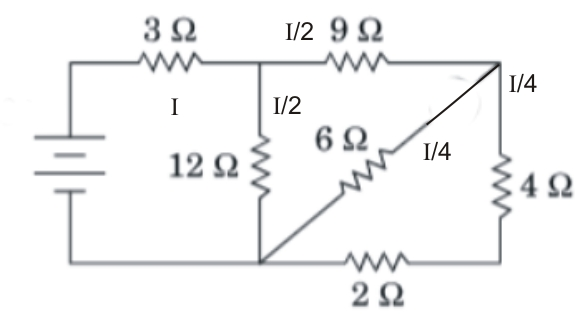from the above diagram, the current through 6 resistance is

Hence, the current shown in the ammeter is 0.25A,

## Related Chapters

### Preparation Products

##### Knockout NEET July 2020

An exhaustive E-learning program for the complete preparation of NEET..

₹ 15999/- ₹ 6999/-
##### Rank Booster NEET 2020

This course will help student to be better prepared and study in the right direction for NEET..

₹ 9999/- ₹ 4999/-
##### Knockout JEE Main July 2020

An exhaustive E-learning program for the complete preparation of JEE Main..

₹ 12999/- ₹ 6999/-
##### Test Series NEET July 2020

Take chapter-wise, subject-wise and Complete syllabus mock tests and get in depth analysis of your test..

₹ 4999/- ₹ 2999/-